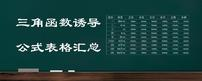# 三角函数归纳公式表汇总，分类归纳有利于系统掌握，非常重要sin(2kπ α)=sinα; 余弦（2kπα）=余弦α； tan(2kπ α)=tanα; cot(2kπ α)=cotα; sec(2kπ α)=secα; csc(2kπα)=cscα。 (k∈Z)

sin(-α)=-sinα； cos(-α)=cosα ;tan(-α)=-tanα;cot(-α)=-cotα;sec(-α)=secα;csc(-α)=-cscα。

p>

sin( π-α)=sinα; cos(π-α)=-cosα； tan(π-α)=-tanα； cot(π-α)=-cotα； sec(π-α)=-secα； -α)=cscα。

sin(2π -α)=sin(-α)=-sinα; 余弦（2π-α）=余弦（-α）=余弦α； tan(2π-α)=tan(-α)=-tanα； cot(2π-α) =cot(-α)=-cotα; sec(2π-α)=sec(-α)=secα； csc(2π-α)=csc(-α)=-cscα。

（1）π/2-α的三角函数值与α的关系：根据三角函数最原始的定义，在一个 直角三角形，两个锐角的三角函数有如下关系：

sin(π/2-α)=cosα； cos(π/2-α)=sinα； tan(π/2-α)=cotα； cot(π/2-α)=tanα； sec(π/ 2-α)=cscα； csc(π/2-α)=secα。

(2) 由式(1)结合 第四组公式，即：

sin(π/2 α)=cosα； cos(π/2α)=-sinα； tan(π/2 α)=-cotα； cot(π/2α)=-tanα； sec (π/2 α)=-cscα； csc(π/2 α)=secα。

(3) 由式(1)结合第二组公式推导出3π/2-α与α的三角函数值的关系，即：

sin(3π/2-α)=-cosα； cos(3π/2-α)=-sinα； tan(3π/2-α)=cotα； cot(3π/2-α)=tanα； sec(3π/2-α)=-cscα； csc(3π/2-α)=-secα。

(4) 3π/2α与α的三角函数值的关系由式(2)结合第二组公式推导出，即：

sin(3π/2α)=- 余弦α; cos(3π/2α)=sinα； tan(3π/2α)=-cotα； cot(3π/2α)=-tanα； sec(3π/2 α )=cscα; csc(3π/2 α)=-secα。